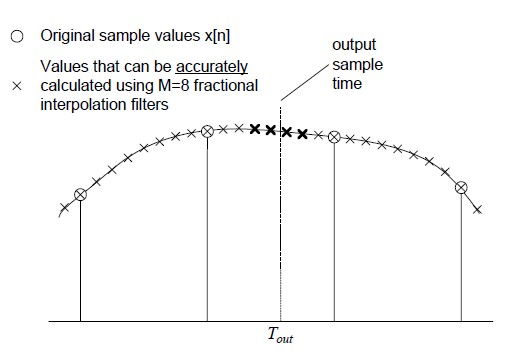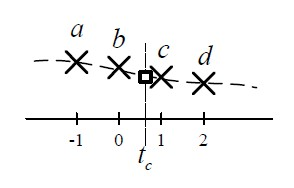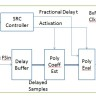# A Practical Design of Digital Fractional Sample Rate Conversion

Make it to the Right and Larger Audience

Blog

# A Practical Design of Digital Fractional Sample Rate Conversion

Sample rate conversion (SRC) is the process of converting a discrete time singal x[n] sampled at a rate FSin to another signal y[m] sampled at another rate FSout.

There are lots of researches done in this area. Here we talk about a practial digital design and our discussions are based on two good papers.

1.  An Efficient Async Sampling-Rate Conversin Algo from Multi-Channel Audio Applications from Paul Beckmann and Timothy Stilson of Analog Inc.
2. An Efficient ALgo for Sample Rate Conversion from CD to DAT  from Kannan Rajamani, Yhean-Sen Lai and C.W. Farrow.As shown above, paper 1 first upsamples x[n] by 8 which can be accurately calculted and then calculates y[m]. Suppose we’d like to calculate an output value at the time indicated by the vertical dashed line, since it doesn’t line up with 8x samples, we need to estimate the value of y[m] based on surrounding 8x samples. Paper 1 uses 2 samples to the left and 2 samples to the right to estimate as shown below.Denote output sample time as Tout. Let’s decompose Tout into integer part N and fractional part t so Tout = N + t and t is less than 1. Let P(t) represent the polynomial used for interpolation. The output y[m] equals P(t) evaluated at t. If we use 3rd order polynomial, P(t) can be expressed as:$P(t)=c_3t^3 + c_2t^2 + c_1t + c_0$
Using 2 samples to the left, sample -1 and sample 0, and 2 samples to the right, sample 1 and sample 2, we have four equations to solve as:$P(-1)=-c_3+c_2-c_1+c_0$$P(0) = c_0$$P(1)=c_3+c_2+c_1+c_0$$P(2)=8c_3+4c_2+2c_1+c_0$

So after resolving four polynomial coefficients, P(t) can be calculated at fractional delay t.

Paper 2 adopts a similar way that a polynomial is used to represent the continuous time waveform around the sampling point and after polynomial coefficients are estimated the sampling value at fractional delay is calculated. The difference is the polynomial is in frequency domain.

But it is not discussed, or to enough detail, how to implement this SRC in a real digital system. For example what clocks are used, for which sub modules, and how to get fractional delay t, etc. In addition, are there other and better ways to represent the polynomial and estimate polynomial coefficients?

In the next, we will talk about these two areas in detail.
1. A practical digital SRC implementation is given with detailed block diagram and discussion. Although no RTL code is given, reader should be able to easily implement it.
2. We also talk about how to use Taylor series and derivative for polynomial approximation and coefficient estimation.

The following is site premium content.
Use points to gain access. You can either purchase points or contribute content and use contribution points to gain access.
Highlights: 2824 words, 7 imagesSenior Engineer
Author brief is empty
Groups:

Tags: